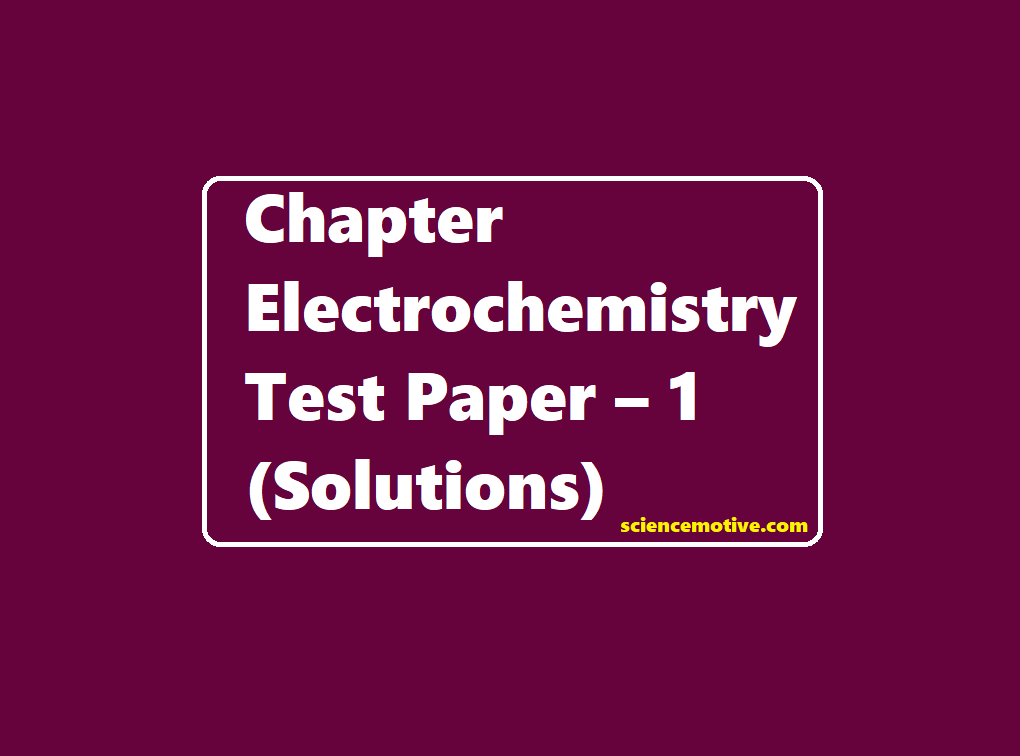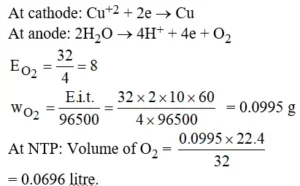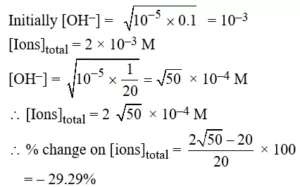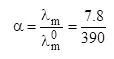# Chapter Electrochemistry Test Paper – 1(Solutions)# Chapter Electrochemistry Test Paper – 1 (Solutions)

Chapter Electrochemistry Test Paper (Solutions)

Ans 1.Ans 2.Ans 3. (A). Any redox reaction would occur spontaneously, if the cell emf is positive.

Ans 4. (C).  2H2O → 2H2(g) + O2(g)
2x                           x
∴ 3x = 0.168
∴ x = 0.056L
VH2= 2x = 0.112L,   VO2= x = 0.056L
11.2L of H2 at STP = 1F
0.112L of H2 at STP = 0.01F
0.056L of O2 at STP = 0.01F
∴ The amount of electricity passed
= 0.01F = 965C

Ans 5. (C). The type of electrode Pb | PbSO4(s) | H2SO4 used in acid storage cell is metal–insoluble salt–anion electrode.

Chapter Electrochemistry Test Paper (Solutions)

Ans 6. (D). At pressure 10–14 atm Pt, H2 | H2O act as a S.H.E.

Ans 7. (A). We know that the reducing power of a metal depends upon its tendency to lose electrons. Thus lower the reduction potential, the more the tendency to get oxidized, and thus more will be the reducing power.
Hence increasing order of reducing power is: Ag < Hg < Cr < Mg < K

Ans 8. (B). The reaction Zn2+ + 2e → Zn has a standard potential of – 0.76 V. This means Zn is a reducing agent.

Ans 9. (B).= 0.02
⇒ Ka (CH3COOH) = Ca2 = 0.04 × (4.8)
⇒ pKa = 6 – log 24 = 6 – 4 × 0.3 = 4.8
⇒ pKb (CH3COO) = 14 – pka = 14 – 4.8 = 9.2

Ans 10. (A). For spontaneous cell reaction in galvanic cell:
Eºcell > 0; ΔGº < 0; Quotient Q < Kc

Ans 11. (A). When an electric current is applied to an aqueous solution of FeCl2 and ZnCl2 reaction at the cathode:
Fe2+(aq) + 2e(aq) → Fe(s) E°red = – 0.44V

Ans 12. (B). The free-energy change and the cell potential have opposite signs.

Ans 13. (D). The given reaction is a reduction reaction.

Ans 14. (D). The standard oxidation potential is
1.14 V – (–0.76 V) = 1.90 V

Ans 15. (A). Cell reaction is:
Zn(s) + Cu2+(aq) → Cu(s) + Zn2+(aq)
Here n = 2
cell = Eºcathode – Eºanode (On the basis of reduction potential)
= + 0.34 – (– 0.76) = 1.10 V
We know that: Wmax = ΔGº = – nFEº
= – (2 mol) × (96500 C mol) × (1.10 V)
= – 212300 J
or Wmax = – 212300 J.

Chapter Electrochemistry Test Paper (Solutions)

Ans 16. (A) A half-cell is prepared by dipping an Ag electrode in a solution containing KCl and some AgCl. Half-cell is used as a cathode during the cell reaction. The quantity of AgCl will decrease.

Ans 17. (D)
Reason: The conductivity of solutions of different electrolytes in the same solvent and at a given temperature differs due to the size and charge of the ions in which they dissociate, the concentration of ions, and the ease with which the ions move under a potential gradient.

Ans 18. (A) and (B)
Reason: Electrolysis of CuSO4 can be represented by two half-cell reactions these occurring at cathode and anode, respectively, as given below:
At cathode: Cu2+ + 2e → Cu(s)
At anode: Cu(s) → Cu2+ + 2e
On dissolution of CuSO4 in water will dissociate as Cu+2, SO4 2–, H+, OH.

Ans 19. (A)
Reason: Copper does not liberate hydrogen from acids because copper lies below hydrogen in electrochemical series. So, copper does not have sufficient electrode potential to liberate elemental hydrogen from compounds in which the oxidation state of hydrogen is +1

Ans 20. (C)
Reason: Ecell is an intensive property and it does not depend upon the number of particles but ΔrG of the cell reaction is an extensive property because this depends upon the number of particles.

Class 12 Chapter Electrochemistry Test Paper – 1